## 74. Datatype ConstructorsUp: Derived Datatypes Next: Subarray Datatype Constructor Previous: Type Constructors with Explicit Addresses

Contiguous The simplest datatype constructor is MPI_TYPE_CONTIGUOUS which allows replication of a datatype into contiguous locations.

 MPI_TYPE_CONTIGUOUS(count, oldtype, newtype) IN count replication count (non-negative integer) IN oldtype old datatype (handle) OUT newtype new datatype (handle)

int MPI_Type_contiguous(int count, MPI_Datatype oldtype, MPI_Datatype *newtype)

MPI_Type_contiguous(count, oldtype, newtype, ierror)
INTEGER, INTENT(IN) :: count
TYPE(MPI_Datatype), INTENT(IN) :: oldtype
TYPE(MPI_Datatype), INTENT(OUT) :: newtype
INTEGER, OPTIONAL, INTENT(OUT) :: ierror
MPI_TYPE_CONTIGUOUS(COUNT, OLDTYPE, NEWTYPE, IERROR)
INTEGER COUNT, OLDTYPE, NEWTYPE, IERROR

newtype is the datatype obtained by concatenating count copies of oldtype. Concatenation is defined using extent as the size of the concatenated copies.

Example Let oldtype have type map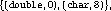with extent 16, and let. The type map of the datatype returned by newtype is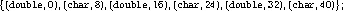i.e., alternating double and char elements, with displacements 0, 8, 16, 24, 32, 40.

In general, assume that the type map of oldtype is { (type0,disp0), ... , (typen-1, dispn-1) } , with extent ex. Then newtype has a type map withentries defined by: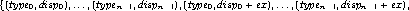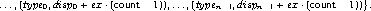Vector The function MPI_TYPE_VECTOR is a more general constructor that allows replication of a datatype into locations that consist of equally spaced blocks. Each block is obtained by concatenating the same number of copies of the old datatype. The spacing between blocks is a multiple of the extent of the old datatype.

 MPI_TYPE_VECTOR(count, blocklength, stride, oldtype, newtype) IN count number of blocks (non-negative integer) IN blocklength number of elements in each block (non-negative integer) IN stride number of elements between start of each block (integer) IN oldtype old datatype (handle) OUT newtype new datatype (handle)

int MPI_Type_vector(int count, int blocklength, int stride, MPI_Datatype oldtype, MPI_Datatype *newtype)

MPI_Type_vector(count, blocklength, stride, oldtype, newtype, ierror)
INTEGER, INTENT(IN) :: count, blocklength, stride
TYPE(MPI_Datatype), INTENT(IN) :: oldtype
TYPE(MPI_Datatype), INTENT(OUT) :: newtype
INTEGER, OPTIONAL, INTENT(OUT) :: ierror
MPI_TYPE_VECTOR(COUNT, BLOCKLENGTH, STRIDE, OLDTYPE, NEWTYPE, IERROR)
INTEGER COUNT, BLOCKLENGTH, STRIDE, OLDTYPE, NEWTYPE, IERROR

Example Assume, again, that oldtype has type mapwith extent 16. A call to MPI_TYPE_VECTOR(2, 3, 4, oldtype, newtype) will create the datatype with type map,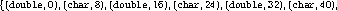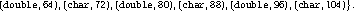That is, two blocks with three copies each of the old type, with a stride of 4 elements (4 · 16 bytes) between the the start of each block.

Example A call to MPI_TYPE_VECTOR(3, 1, -2, oldtype, newtype) will create the datatype,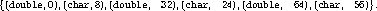In general, assume that oldtype has type map, { (type0,disp0), ..., (typen-1, dispn-1) } , with extent ex. Let bl be the blocklength. The newly created datatype has a type map with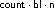entries: { (type0, disp0), ... , (typen-1 , dispn-1), (type0 ,disp0 + ex) , ... , (typen-1 , dispn-1 + ex ), ...,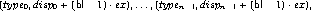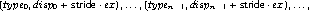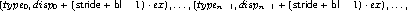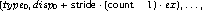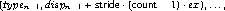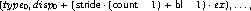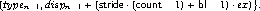A call to MPI_TYPE_CONTIGUOUS(count, oldtype, newtype) is equivalent to a call to MPI_TYPE_VECTOR(count, 1, 1, oldtype, newtype), or to a call to MPI_TYPE_VECTOR(1, count, n, oldtype, newtype), n arbitrary.

Hvector The function MPI_TYPE_CREATE_HVECTOR is identical to MPI_TYPE_VECTOR, except that stride is given in bytes, rather than in elements. The use for both types of vector constructors is illustrated in Section Examples . ( H stands for ``heterogeneous'').

 MPI_TYPE_CREATE_HVECTOR(count, blocklength, stride, oldtype, newtype) IN count number of blocks (non-negative integer) IN blocklength number of elements in each block (non-negative integer) IN stride number of bytes between start of each block (integer) IN oldtype old datatype (handle) OUT newtype new datatype (handle)

int MPI_Type_create_hvector(int count, int blocklength, MPI_Aint stride, MPI_Datatype oldtype, MPI_Datatype *newtype)

MPI_Type_create_hvector(count, blocklength, stride, oldtype, newtype, ierror)
INTEGER, INTENT(IN) :: count, blocklength
TYPE(MPI_Datatype), INTENT(IN) :: oldtype
TYPE(MPI_Datatype), INTENT(OUT) :: newtype
INTEGER, OPTIONAL, INTENT(OUT) :: ierror
MPI_TYPE_CREATE_HVECTOR(COUNT, BLOCKLENGTH, STRIDE, OLDTYPE, NEWTYPE, IERROR)
INTEGER COUNT, BLOCKLENGTH, OLDTYPE, NEWTYPE, IERROR

Assume that oldtype has type map, { (type0,disp0), ..., (typen-1, dispn-1) } , with extent ex. Let bl be the blocklength. The newly created datatype has a type map with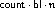entries: { (type0, disp0), ... , (typen-1 , dispn-1), (type0 ,disp0 + ex) , ... , (typen-1 , dispn-1 + ex ), ...,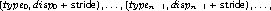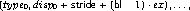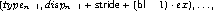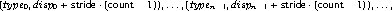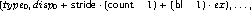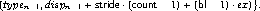Indexed The function MPI_TYPE_INDEXED allows replication of an old datatype into a sequence of blocks (each block is a concatenation of the old datatype), where each block can contain a different number of copies and have a different displacement. All block displacements are multiples of the old type extent.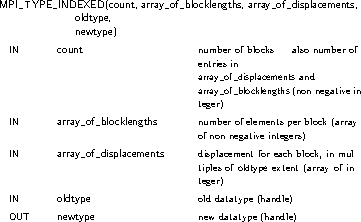int MPI_Type_indexed(int count, const int array_of_blocklengths[], const int array_of_displacements[], MPI_Datatype oldtype, MPI_Datatype *newtype)

MPI_Type_indexed(count, array_of_blocklengths, array_of_displacements, oldtype, newtype, ierror)
INTEGER, INTENT(IN) :: count, array_of_blocklengths(count),
array_of_displacements(count)
TYPE(MPI_Datatype), INTENT(IN) :: oldtype
TYPE(MPI_Datatype), INTENT(OUT) :: newtype
INTEGER, OPTIONAL, INTENT(OUT) :: ierror
MPI_TYPE_INDEXED(COUNT, ARRAY_OF_BLOCKLENGTHS, ARRAY_OF_DISPLACEMENTS, OLDTYPE, NEWTYPE, IERROR)
INTEGER COUNT, ARRAY_OF_BLOCKLENGTHS(*), ARRAY_OF_DISPLACEMENTS(*),
OLDTYPE, NEWTYPE, IERROR

Example Let oldtype have type mapwith extent 16. Let B = (3, 1) and let D = (4, 0). A call to MPI_TYPE_INDEXED(2, B, D, oldtype, newtype) returns a datatype with type map,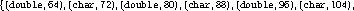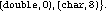That is, three copies of the old type starting at displacement 64, and one copy starting at displacement 0.

In general, assume that oldtype has type map, { (type0,disp0), ..., (typen-1, dispn-1) } , with extent ex. Let B be the array_of_blocklengths argument and D be the array_of_displacements argument. The newly created datatype has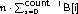entries: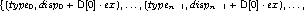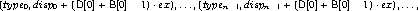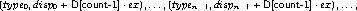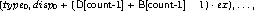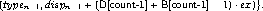A call to MPI_TYPE_VECTOR(count, blocklength, stride, oldtype, newtype) is equivalent to a call to MPI_TYPE_INDEXED(count, B, D, oldtype, newtype) where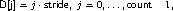and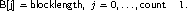Hindexed The function MPI_TYPE_CREATE_HINDEXED is identical to MPI_TYPE_INDEXED, except that block displacements in array_of_displacements are specified in bytes, rather than in multiples of the oldtype extent.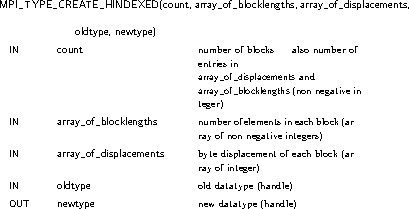int MPI_Type_create_hindexed(int count, const int array_of_blocklengths[], const MPI_Aint array_of_displacements[], MPI_Datatype oldtype, MPI_Datatype *newtype)

MPI_Type_create_hindexed(count, array_of_blocklengths, array_of_displacements, oldtype, newtype, ierror)
INTEGER, INTENT(IN) :: count, array_of_blocklengths(count)
array_of_displacements(count)
TYPE(MPI_Datatype), INTENT(IN) :: oldtype
TYPE(MPI_Datatype), INTENT(OUT) :: newtype
INTEGER, OPTIONAL, INTENT(OUT) :: ierror
MPI_TYPE_CREATE_HINDEXED(COUNT, ARRAY_OF_BLOCKLENGTHS, ARRAY_OF_DISPLACEMENTS, OLDTYPE, NEWTYPE, IERROR)
INTEGER COUNT, ARRAY_OF_BLOCKLENGTHS(*), OLDTYPE, NEWTYPE, IERROR

Assume that oldtype has type map, { (type0,disp0), ..., (typen-1, dispn-1) } , with extent ex. Let B be the array_of_blocklengths argument and D be the array_of_displacements argument. The newly created datatype has a type map withentries: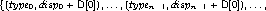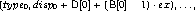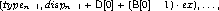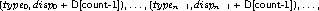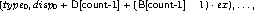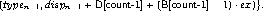Indexed_block This function is the same as MPI_TYPE_INDEXED except that the blocklength is the same for all blocks. There are many codes using indirect addressing arising from unstructured grids where the blocksize is always 1 (gather/scatter). The following convenience function allows for constant blocksize and arbitrary displacements.

 MPI_TYPE_CREATE_INDEXED_BLOCK(count, blocklength, array_of_displacements, oldtype, newtype) IN count length of array of displacements (non-negative integer) IN blocklength size of block (non-negative integer) IN array_of_displacements array of displacements (array of integer) IN oldtype old datatype (handle) OUT newtype new datatype (handle)

int MPI_Type_create_indexed_block(int count, int blocklength, const int array_of_displacements[], MPI_Datatype oldtype, MPI_Datatype *newtype)

MPI_Type_create_indexed_block(count, blocklength, array_of_displacements, oldtype, newtype, ierror)
INTEGER, INTENT(IN) :: count, blocklength,
array_of_displacements(count)
TYPE(MPI_Datatype), INTENT(IN) :: oldtype
TYPE(MPI_Datatype), INTENT(OUT) :: newtype
INTEGER, OPTIONAL, INTENT(OUT) :: ierror
MPI_TYPE_CREATE_INDEXED_BLOCK(COUNT, BLOCKLENGTH, ARRAY_OF_DISPLACEMENTS, OLDTYPE, NEWTYPE, IERROR)
INTEGER COUNT, BLOCKLENGTH, ARRAY_OF_DISPLACEMENTS(*), OLDTYPE,
NEWTYPE, IERROR

Hindexed_block The function MPI_TYPE_CREATE_HINDEXED_BLOCK is identical to MPI_TYPE_CREATE_INDEXED_BLOCK, except that block displacements in array_of_displacements are specified in bytes, rather than in multiples of the oldtype extent.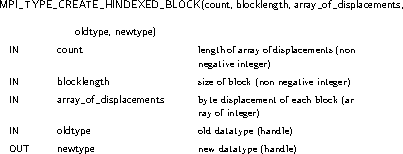int MPI_Type_create_hindexed_block(int count, int blocklength, const MPI_Aint array_of_displacements[], MPI_Datatype oldtype, MPI_Datatype *newtype)

MPI_Type_create_hindexed_block(count, blocklength, array_of_displacements, oldtype, newtype, ierror)
INTEGER, INTENT(IN) :: count, blocklength
array_of_displacements(count)
TYPE(MPI_Datatype), INTENT(IN) :: oldtype
TYPE(MPI_Datatype), INTENT(OUT) :: newtype
INTEGER, OPTIONAL, INTENT(OUT) :: ierror
MPI_TYPE_CREATE_HINDEXED_BLOCK(COUNT, BLOCKLENGTH, ARRAY_OF_DISPLACEMENTS, OLDTYPE, NEWTYPE, IERROR)
INTEGER COUNT, BLOCKLENGTH, OLDTYPE, NEWTYPE, IERROR

Struct MPI_TYPE_CREATE_STRUCT is the most general type constructor. It further generalizes MPI_TYPE_CREATE_HINDEXED in that it allows each block to consist of replications of different datatypes.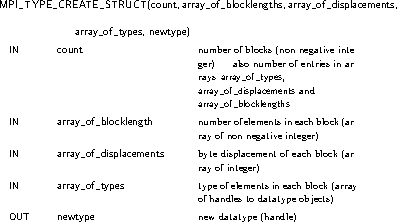int MPI_Type_create_struct(int count, const int array_of_blocklengths[], const MPI_Aint array_of_displacements[], const MPI_Datatype array_of_types[], MPI_Datatype *newtype)

MPI_Type_create_struct(count, array_of_blocklengths, array_of_displacements, array_of_types, newtype, ierror)
INTEGER, INTENT(IN) :: count, array_of_blocklengths(count)
array_of_displacements(count)
TYPE(MPI_Datatype), INTENT(IN) :: array_of_types(count)
TYPE(MPI_Datatype), INTENT(OUT) :: newtype
INTEGER, OPTIONAL, INTENT(OUT) :: ierror
MPI_TYPE_CREATE_STRUCT(COUNT, ARRAY_OF_BLOCKLENGTHS, ARRAY_OF_DISPLACEMENTS, ARRAY_OF_TYPES, NEWTYPE, IERROR)
INTEGER COUNT, ARRAY_OF_BLOCKLENGTHS(*), ARRAY_OF_TYPES(*), NEWTYPE,
IERROR

Example Let type1 have type map,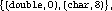with extent 16. Let B = (2, 1, 3), D = (0, 16, 26), and T = (MPI_FLOAT, type1, MPI_CHAR). Then a call to MPI_TYPE_CREATE_STRUCT(3, B, D, T, newtype) returns a datatype with type map,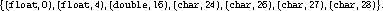That is, two copies of MPI_FLOAT starting at 0, followed by one copy of type1 starting at 16, followed by three copies of MPI_CHAR, starting at 26. (We assume that a float occupies four bytes.)

In general, let T be the array_of_types argument, where T[i] is a handle to, typemapi = { (type0i , disp0i ) , ... , (typeni-1i , dispni-1i ) } , with extent exi. Let B be the array_of_blocklength argument and D be the array_of_displacements argument.Let c be the count argument. Then the newly created datatype has a type map with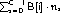entries: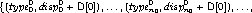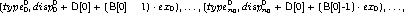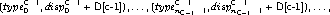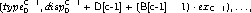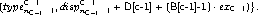A call to MPI_TYPE_CREATE_HINDEXED(count, B, D, oldtype, newtype) is equivalent to a call to MPI_TYPE_CREATE_STRUCT(count, B, D, T, newtype), where each entry of T is equal to oldtype.Up: Derived Datatypes Next: Subarray Datatype Constructor Previous: Type Constructors with Explicit Addresses